Robotics 2
Review
QuizQuestion 2:  For the kinematic diagram in Question 1, what is the rotation matrix from frame 1 to frame 2?
Question 3:  For the kinematic diagram in Question 1, what is the displacement vector from frame 0 to frame 1?
Question 4:  For the kinematic diagram in Question 1, what is the displacement vector from frame 1 to frame 2?
Question 5:  For the kinematic diagram in Question 1, what is the homogeneous transformation matrix from frame 2 to frame 3?
Question 6:  Shown here is a homogeneous transformation matrix.  Which picture of two frames correctly represents this matrix?
Question 8:  When finding the inverse kinematics equations, what should be on the left side of the equations, and what should be on the right side?
Question 9:  Shown here is a two-degree-of-freedom manipulator.  When the link lengths are as shown, what value of Theta 1 will get the end-effector to the specified X, Y, Z location?  Round your answer to two decimal places.
Question 10: For the setup in Question 9, what value of Theta 2 will get the end-effector to the specified X, Y, Z location?  Round your answer to two decimal places.
Question 1: Shown here is a labeled kinematic diagram.  Which of these matrices is the rotation matrix from frame 0 to frame1?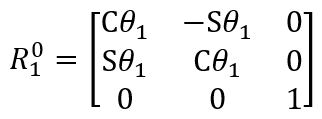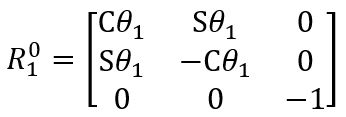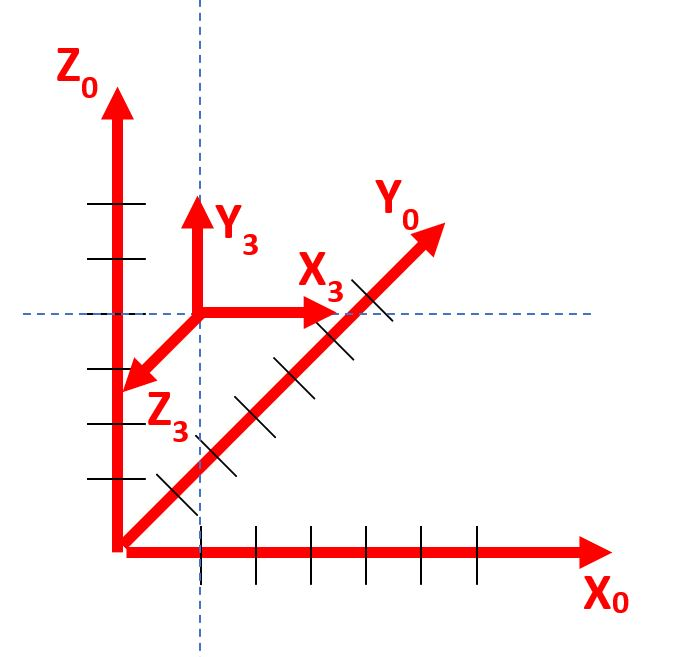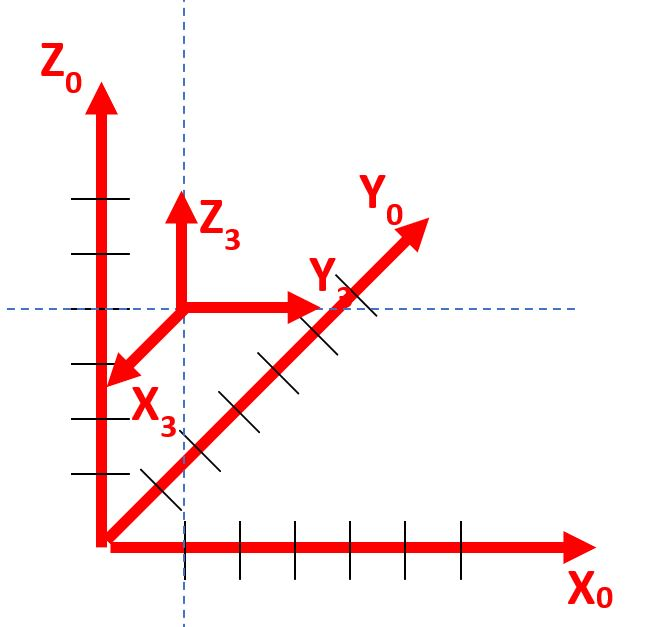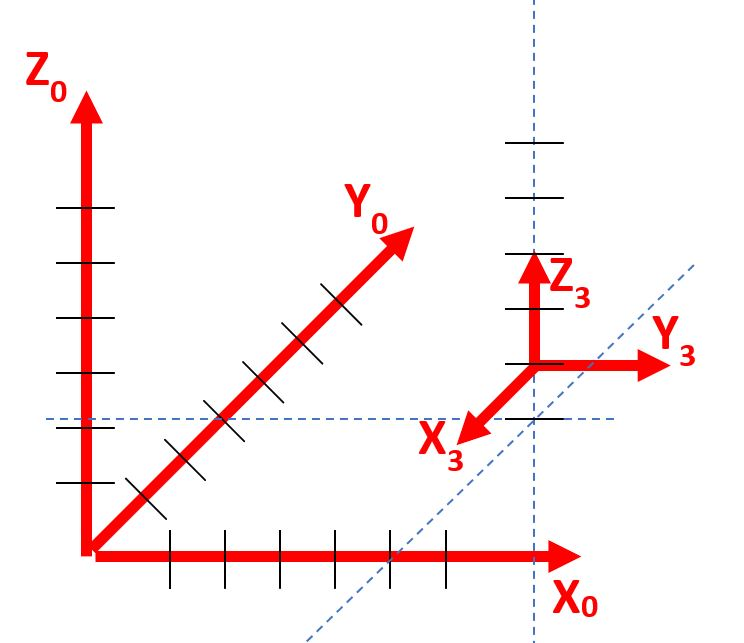Question 7:  Shown here is a picture of two frames.  Which homogeneous transformation matrix is correct?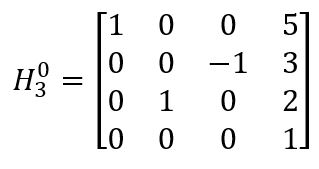Left Side: unknown quantities (including the position of the end-effector)
Right Side: known quantities (including the joint variables)
Left Side: position and rotation of the end-effector
Right Side: link lengths
Left Side: joint variables
Right Side: known quantities (including the position of the end-effector)
Left Side: joint variables
Right Side: unknown quantities (including the link lengths)degrees
Theta 1 =
Theta 2 =
degrees